# 函数参数的类型1148-张同学

## 参数

#### 位置参数

// An highlighted block
def test01(a,b,c):
d=a*b*c
print(d)

#test01(20,30)  #形参和实参的个数需要对应，不然会出错
test01(20,30,40)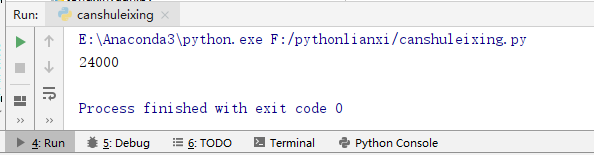#### 默认值参数

// An highlighted block
def test01(a,b,c=40,d=50):
print(a,b,c,d)

test01(20,30)     #这时传递的是a，b的值
test01(20,30,70)  #这时传递的是a，b，c的值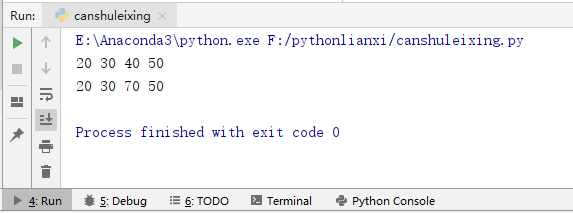#### 命名参数

// An highlighted block
def test01(a,b,c):
print(a,b,c)

test01(8,9,10)     #位置参数
test01(b=9,a=8,c=10)  #命令参数，位置可以改变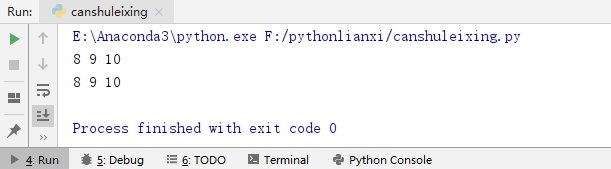#### 可变参数

1. *param “元组”
2. **param “字典”
// An highlighted block
def test01(a,b,*c):
print(a,b,c)

def test02(a,b,**c):
print(a,b,c)

test01(8,9,100,200)     #元组
test02(8,9,name='一个小白的日常',age=24)     #字典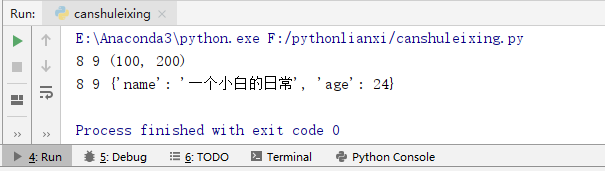#### lambda表达式和匿名函数

arg1,arg2,arg3为函数的参数。
<表达式>相当于函数体，运行结果：表达式的运算结果。

// An highlighted block
f=lambda a,b,c:a+b+c
print(f)
print(f(2,3,4))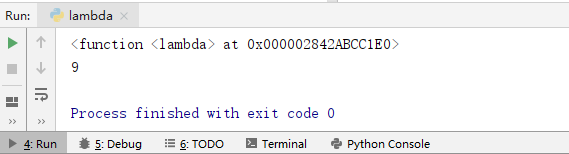#### eval()函数

source：一个python表达式或函数compile()返回的代码对象。
globals：可选，必须是dictionary.
locals：可选，任意映射对象。

// An highlighted block
s="print('abc')"
eval(s)    #执行输出语句
s="print('一个小白的日常')"
eval(s)    #当参数变得时候，也会跟着改变

a=10
b=20
c=eval("a*b")
print(c)        #执行乘法运算

dict1=dict(a=100,b=200)
d=eval("a*b",dict1)
print(d)           #执行元组的参数的乘法运算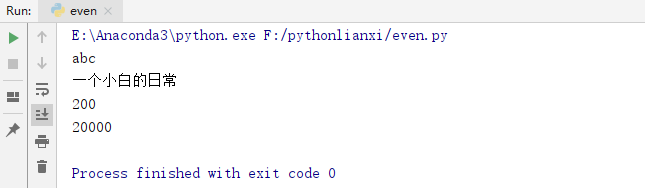#### 递归函数

1. 终止条件：表示递归什么时候结束，一般用于返回值，不再调用自己。
2. 递归步骤：把第n步的值和第n-1步相关联。

// An highlighted block
def test01(n):
print("test01:",n,end="/t")
if n==0:         #当n为0时，整个调用停止
print("over")
else:             #控制调用次数n在一定范围就结束
test01(n-1)
print("test01***",n,end="/t")     #不参与递归调用，就是普通调用

test01(5)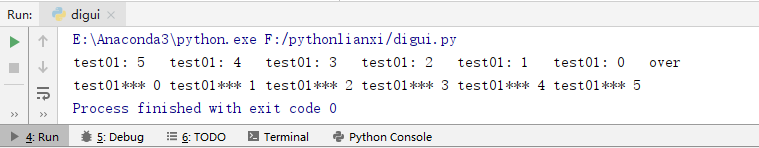## 练习：递归实现阶乘

// An highlighted block
'''练习阶乘'''
def factional(n):
if n==1:
return 1    #求1的阶乘时，直接返回1
else:
return n*factional(n-1)          #不为1时，则通过调用求阶乘

for i in range(1,6):
print(i,"!=",factional(i),end='/t')              #1-5的阶乘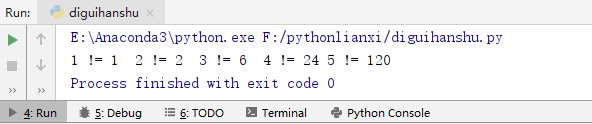### 评论 抢沙发### 觉得文章有用就打赏一下文章作者

#### 支付宝扫一扫打赏#### 微信扫一扫打赏Vieu3.3主题Q Q 登 录